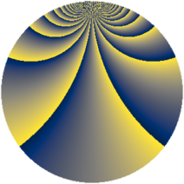# Properties

 Label 1380.2.bvLevel $1380$ Weight $2$ Character orbit 1380.bv Rep. character $\chi_{1380}(83,\cdot)$ Character field $\Q(\zeta_{44})$ Dimension $5600$ Sturm bound $576$

# Related objects

## Defining parameters

 Level: $$N$$ $$=$$ $$1380 = 2^{2} \cdot 3 \cdot 5 \cdot 23$$ Weight: $$k$$ $$=$$ $$2$$ Character orbit: $$[\chi]$$ $$=$$ 1380.bv (of order $$44$$ and degree $$20$$) Character conductor: $$\operatorname{cond}(\chi)$$ $$=$$ $$1380$$ Character field: $$\Q(\zeta_{44})$$ Sturm bound: $$576$$

## Dimensions

The following table gives the dimensions of various subspaces of $$M_{2}(1380, [\chi])$$.

Total New Old
Modular forms 5920 5920 0
Cusp forms 5600 5600 0
Eisenstein series 320 320 0

## Trace form

 $$5600q - 36q^{6} + O(q^{10})$$ $$5600q - 36q^{6} - 44q^{10} - 6q^{12} - 72q^{13} - 80q^{16} - 10q^{18} - 88q^{21} - 72q^{25} - 44q^{28} - 22q^{30} - 44q^{33} - 68q^{36} - 88q^{37} - 44q^{40} - 22q^{42} - 56q^{46} + 22q^{48} + 112q^{52} - 44q^{57} - 52q^{58} - 22q^{60} - 176q^{61} - 44q^{66} - 80q^{70} + 14q^{72} - 72q^{73} - 88q^{76} - 42q^{78} + 8q^{81} - 12q^{82} - 72q^{85} - 44q^{88} - 440q^{90} - 152q^{93} - 36q^{96} - 88q^{97} + O(q^{100})$$

## Decomposition of $$S_{2}^{\mathrm{new}}(1380, [\chi])$$ into newform subspaces

The newforms in this space have not yet been added to the LMFDB.